Charging and discharging of charge and discharge capacitors
2022-12-20

## What is a Capacitor?

A capacitor is a device that is used for storing electrical energy in an electric field. A capacitor has two conductors that are close, but isolated from each other by an insulator or non-conductive region. This insulator is also known as dielectric. The conductors are usually a thin metal; and the insulator can be made of glass, air, film, ceramic, and other materials. Capacitors are mainly used in energy storage, such as the digital circuits of a computer. They store the electrical energy so that information is not lost during an electrical outage. Other applications of capacitors include:

1. Power conditioning

2. Signal coupling or decoupling

3. Electronic noise filtering

4. Remote sensing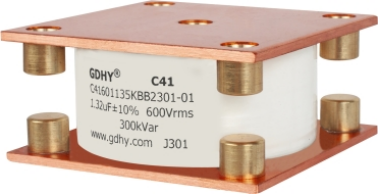A capacitor has two plates: a positive plate and a negative plate. As electrical current flows through the electrical circuit, the capacitor collects energy at the positive plate. An equal amount of electrical charge flows from the negative plate simultaneously, maintaining equal charges at both plates. The capacitor holds the energy that was collected when the electrical circuit is switched off.

Capacitance (C) is the ability of a system to store electrical charges. It is the ratio of the change in an electric charge in a system to the corresponding change in its electric potential. The capacitance of a capacitor is measured in units called farads (F).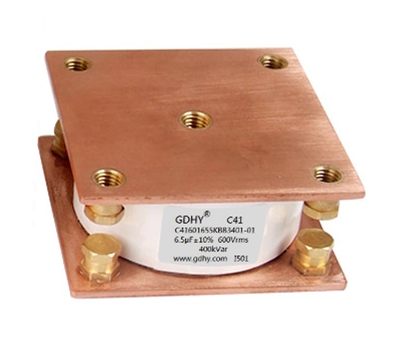Charging and Discharging of a Capacitor

When a capacitor is connected to a direct current (DC) circuit, charging or discharging may occur. Charging refers to the situation where there is an increase in potential difference, while both conducting plates get an equal and opposite charge. Direct current is a unidirectional flow of electric charge. The capacitor is fully charged when the voltage of the power supply is equal to that at the capacitor terminals. This is called capacitor charging; and the charging phase is over when current stops flowing through the electrical circuit.

When the power supply is removed from the capacitor, the discharging phase begins. During discharging, there is a constant reduction in the voltage between the two plates until it reaches zero.

The time constant (T) is the product of the resistance and the capacitance. It reflects the rate at which a capacitor charges and discharges. The time constant is proportional to the resistance and capacitance. If the resistance and capacitance is small, then the time constant will also be small. A small time constant also means that the capacitor will charge and discharge at a faster rate.

Time constant equation: T=RC, where T is the time constant measured in seconds (s), R is the resistance measured in ohm (ohm), and C is the capacitance measured in Farads (F).

## Charging graphs:

When a Capacitor is connected to a circuit with Direct Current (DC) source, two processes, which are called "charging" and "discharging" the Capacitor, will happen in specific conditions.

In Figure 1, the Capacitor is connected to the DC Power Supply and Current flows through the circuit. Both Plates get the equal and opposite charges and an increasing Potential Difference, vc, is created while the Capacitor is charging. Once the Voltage at the terminals of the Capacitor, vc, is equal to the Power Supply Voltage, vc = V, the Capacitor is fully charged and the Current stops flowing through the circuit, the Charging Phase is over.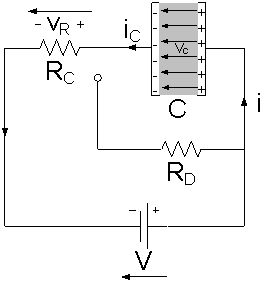Figure 1: The capacitor is charging

When a capacitor charges, electrons flow onto one plate and move off the other plate. This process will be continued unitl the potential difference across the capacitor is equal to the potential difference across the battery. Because the current changes throughout charging, the rate of flow of charge will not be linear.

At the start, the current will be at its highest but will gradually decrease to zero. The following graphs summarise capacitor charge. The potential difference and charge graphs look the same because they are proportional.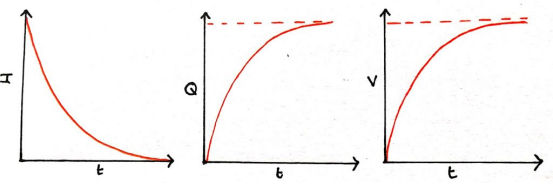You can also see that the gradient of the charge-time graph is related to the current-time graph. This is because the gradient of the charge-time graph is charge / time = current.

Discharging graphs:

A Capacitor is equivalent to an Open-Circuit to Direct Current, R = ∞, because once the Charging Phase has finished, no more Current flows through it. The Voltage vc on a Capacitor cannot change abruptly.

When the Capacitor disconnected from the Power Supply, the Capacitor is discharging through the Resistor RD and the Voltage between the Plates drops down gradually to zero, vc = 0, Figure 2.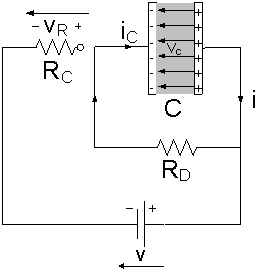Figure 2: The capacitor is discharging

When a capacitor is discharged, the current will be highest at the start. this will gradually decrease until reaching 0, when the current reaches zero, the capacitor is fully discharged as there is no charge stored across it.

The rate of decrease of the potential difference and the charge will again be proportional to the value of the current. This time all of the graphs will have the same shape: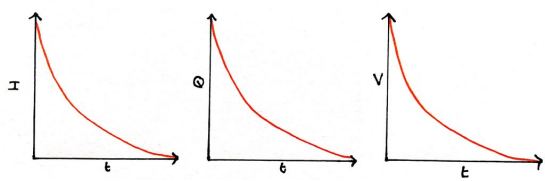## Resistance and capacitance:

The rate at which a capacitor charges or discharges will depend on the resistance of the circuit. Resistance reduces the current which can flow through a circuit so the rate at which the charge flows will be reduced with a higher resistance. This means increasing the resistance will increase the time for the capacitor to charge or discharge. It won't affect the final pd or the total charge stored at the end.

The other factor which affects the rate of charge is the capacitance of the capacitor. A higher capacitance means that more charge can be stored, it will take longer for all this charge to flow to the capacitor.

## Time constant:

The product of Resistance R and Capacitance C is called the Time Constant τ, which characterizes the rate of charging and discharging of a Capacitor, Figure 3.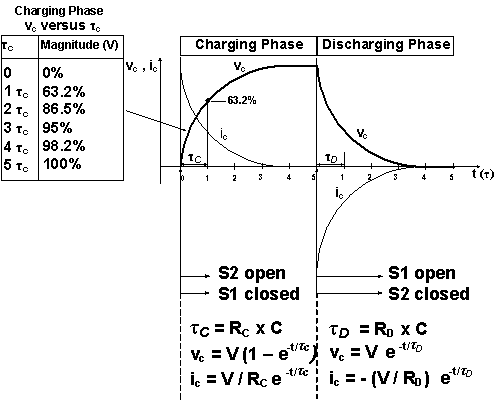Figure 3: The Voltage vc and the Current iC during the Charging Phase and Discharging Phase

The smaller the Resistance or the Capacitance, the smaller the Time Constant, the faster the charging and the discharging rate of the Capacitor, and vice versa.

Capacitors are found in almost all electronic circuits. They can be used as a fast battery. For example, a Capacitor is a storehouse of energy in photoflash unit that releases the energy quickly during short period of the flash.

The time constant is the time it takes for the charge on a capacitor to decrease to(about 37%). The two factors which affect the rate at which charge flows are resistance and capacitance. This means that the following equation can be used to find the time constant:τ = C R

Where τ is the time constant, C  is capacitance and R is resistance

The discharge of a capacitor is exponential, the rate at which charge decreases is proportional to the amount of charge which is left. Like with radioactive decay and half life, the time constant will be the same for any point on the graph: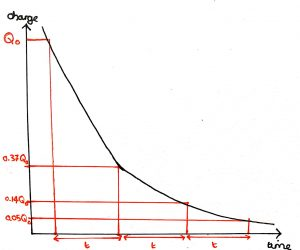Each time the charge on the capacitor is reduced by 37%, it takes the same amount of time. This time taken is the time constant.

Example: Find the time constant for a capacitor with capacitance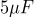in a circuit with a resistance of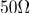: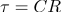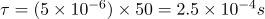## Equations for discharge:

The time constant we have used above can be used to make the equations we need for the discharge of a capacitor. A general equation for exponential decay is: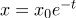For the equation of capacitor discharge, we put in the time constant, and then substitute x for Q, V or I: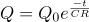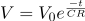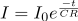Where: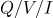is charge/pd/current at time t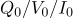is charge/pd/current at start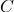is capacitance and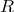is the resistance

When the time, t, is equal to the time constant the equation for charge becomes: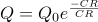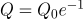This means that the charge is nowtimes the original or 37%.

Example: A capacitor with a capacitance of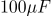is fully charged, holding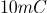of charge. It is discharged through a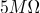resistor. Calculate the charge after 50 seconds and the time for the potential difference to drop below 12V:Substitute in the time 50s, C, R and the initial charge, Q0: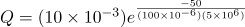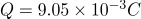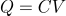so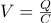Initial potential difference is therefore: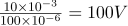Substitute in what we know, putting 12V as the pd at time t: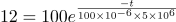Divide by 100: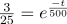Take logs of each side: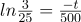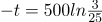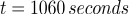## Equations for charging:

The charge after a certain time charging can be found using the following equations: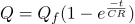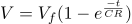Where:

Q/V/I is charge/pd/current at time t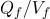is maximum final charge/pd

C is capacitance and R is the resistance

## Graphical analysis:

We can plot an exponential graph of charging and discharging a capacitor, as shown before. However, by manipulating the equation for discharging, we can produce a straight line graph:Take logs of both sides: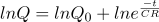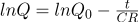This is in the form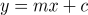where:

1. y is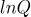2. x is t

3. m is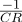4. c is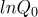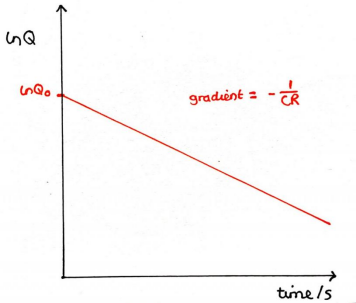## Investigating charge and discharge of capacitors:

An experiment can be carried out to investigate how the potential difference and current change as capacitors charge and discharge. The method is given below:

1. A circuit is set up as shown below, using a capacitor with high capacitance and a resistor of high resistance slows down the changes (higher time constant) so it is easier to measure: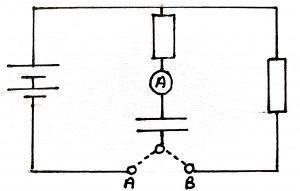2. The switch is closed at A and the capacitor begins to charge

3. Record the current and pd every 20 seconds

4. Once the capacitor is fully charged, close the switch at B and measure the current and pd every 20 seconds.

5. Plot graphs for the current and pd as the capacitor is first charged then discharged.

RECOMMENDED FOR YOU
GDHY Revolutionizing Capacitor Design to Enhance Performance and Efficiency in Small Welding and Quenching Equipment
Precautions for using film capacitors
Start capacitor in AC Induction Motor
How does metallized polyester film capacitors work in the application?
How to chose water cooled resonant capacitor ?
What is an axial film capacitor?
What is a plastic film capacitor?
IGBT Snubber Capacitors Selection Guide
What is a metallized polyester film capacitor?
What is a coupling capacitor and a bypass capacitor?
Get In Touch With Us
If you contact us now for more details, you can take samples for free. Our service team will get back to you with in 24 hours normally !